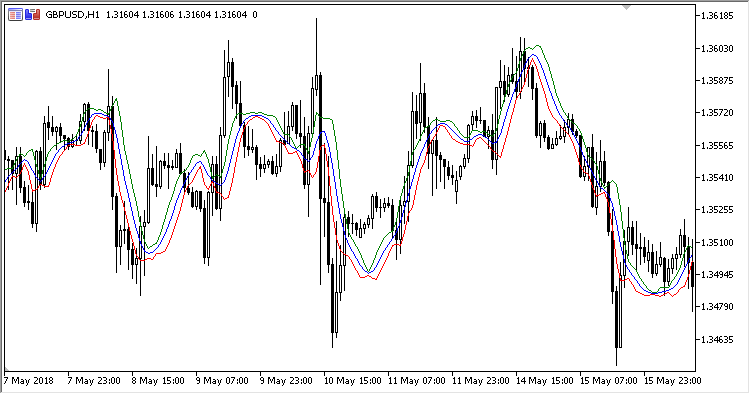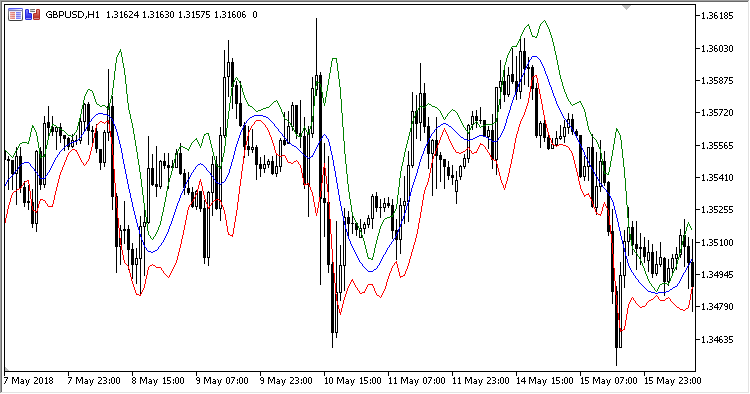Interesting script?
So post a link to it -
let others appraise it

You liked the script? Try it in the MetaTrader 5 terminal# Standard_Error_Bands - indicator for MetaTrader 5

Views:
1198
Rating:
Published:
2018.10.25 18:13

Standard Error Bands indicator - Smoothed linear regression line: 21-period linear regression curve smoothed by a three-period simple moving average. Upper channel line - linear regression line plus 2 standard errors. Lower channel line is a linear regression line minus 2 standard errors.

The indicator was described by John Andersen in "Stock and Commodities" magazine in September 1996.

It has four inputs:

• Regression period - linear regression period
• Smoothing period
• Multiplier - standard deviation ratio
• Applied price

Calculation:

```Central = SMA(LReg,Smoothing period)
Top    = Central + Multiplier * Deviation
Bottom = Central - Multiplier * Deviation
```

where:

```Deviation = StdDev(LReg,Smoothing period)
LReg - linear regression at 'Applied price' with the 'Regression period' calculation period
```Translated from Russian by MetaQuotes Software Corp.
Original code: https://www.mql5.com/ru/code/22364Volatility_Stop

Volatility Stop indicatorVolatility_Stop_Oscillator

Volatility Stop oscillatorSSSAR

Single Stream SAR indicatorMurreys_Math_Oscillator

Murreys Math Oscillator